Updating search results...

# 28 Results

View
Selected filters:
• numeracy-6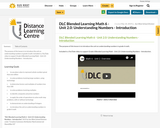Conditional Remix & Share Permitted
CC BY-NC-SA
Rating
0.0 stars

The purpose of this lesson is to introduce the unit on understanding numbers in grade 6 math.

Included is a YouTube video to support Grade 6 Blended Learning Math - Unit 2.0: Understanding Numbers - Introduction.

Subject:
Math
Material Type:
Activity/Lab
Homework/Assignment
Lesson
Provider:
Sun West Distance Learning Centre (DLC)
05/10/2019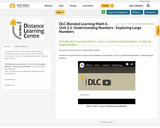Conditional Remix & Share Permitted
CC BY-NC-SA
Rating
0.0 stars

The purpose of this lesson is to explore large numbers.

Included is a YouTube video to support Grade 6 Blended Learning Math - Unit 2.1: Understanding Numbers - Exploring Large Numbers.

Subject:
Math
Material Type:
Activity/Lab
Homework/Assignment
Lesson
Provider:
Sun West Distance Learning Centre (DLC)
05/10/2019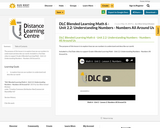Conditional Remix & Share Permitted
CC BY-NC-SA
Rating
0.0 stars

The purpose of this lesson is to explore how we use numbers to understand and describe our world.

Included is a YouTube video to support Grade 6 Blended Learning Math - Unit 2.2: Understanding Numbers - Numbers All Around Us .

Subject:
Math
Material Type:
Activity/Lab
Homework/Assignment
Lesson
Provider:
Sun West Distance Learning Centre (DLC)
05/14/2019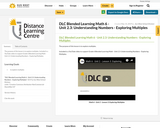Conditional Remix & Share Permitted
CC BY-NC-SA
Rating
0.0 stars

The purpose of this lesson is to explore multiples.

Included is a YouTube video to support Grade 6 Blended Learning Math - Unit 2.3: Understanding Numbers - Exploring Multiples.

Subject:
Math
Material Type:
Activity/Lab
Homework/Assignment
Lesson
Provider:
Sun West Distance Learning Centre (DLC)
05/14/2019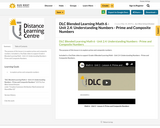Conditional Remix & Share Permitted
CC BY-NC-SA
Rating
0.0 stars

The purpose of this lesson is to explore prime and composite numbers.

Included is a YouTube video to support Grade 6 Blended Learning Math - Unit 2.4: Understanding Numbers - Prime and Composite Numbers.

Subject:
Math
Material Type:
Activity/Lab
Homework/Assignment
Lesson
Provider:
Sun West Distance Learning Centre (DLC)
05/14/2019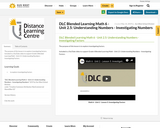Conditional Remix & Share Permitted
CC BY-NC-SA
Rating
0.0 stars

The purpose of this lesson is to explore investigating factors.

Included is a YouTube video to support Grade 6 Blended Learning Math - Unit 2.5: Understanding Numbers - Investigating Factors.

Subject:
Math
Material Type:
Activity/Lab
Homework/Assignment
Lesson
Provider:
Sun West Distance Learning Centre (DLC)
05/14/2019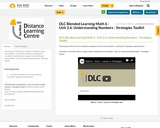Conditional Remix & Share Permitted
CC BY-NC-SA
Rating
0.0 stars

The purpose of this lesson is to explore strategies to solve word problems - specifically "keeping an organized list".

Included is a YouTube video to support Grade 6 Blended Learning Math - Unit 2.6: Understanding Numbers - Strategies Toolkit.

Subject:
Math
Material Type:
Activity/Lab
Homework/Assignment
Lesson
Provider:
Sun West School Division
05/14/2019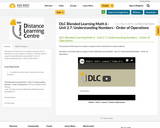Conditional Remix & Share Permitted
CC BY-NC-SA
Rating
0.0 stars

The purpose of this lesson is to explore using the order of operations to solve problems.

Included is a YouTube video to support Grade 6 Blended Learning Math - Unit 2.7: Understanding Numbers - Order of Operations.

Subject:
Math
Material Type:
Activity/Lab
Homework/Assignment
Lesson
Provider:
Sun West Distance Learning Centre (DLC)
05/14/2019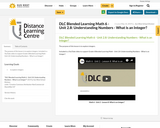Conditional Remix & Share Permitted
CC BY-NC-SA
Rating
0.0 stars

The purpose of this lesson is to explore integers.

Included is a YouTube video to support Grade 6 Blended Learning Math - Unit 2.8: Understanding Numbers - What is an Integer?.

Subject:
Math
Material Type:
Activity/Lab
Homework/Assignment
Lesson
Provider:
Sun West Distance Learning Centre (DLC)
05/14/2019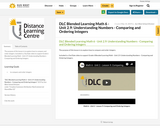Conditional Remix & Share Permitted
CC BY-NC-SA
Rating
0.0 stars

The purpose of this lesson is to explore how to compare and order integers.

Included is a YouTube video to support Grade 6 Blended Learning Math - Unit 2.9: Understanding Numbers - Comparing and Ordering Integers.

Subject:
Math
Material Type:
Activity/Lab
Homework/Assignment
Lesson
Provider:
Sun West Distance Learning Centre (DLC)
05/14/2019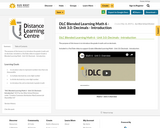Conditional Remix & Share Permitted
CC BY-NC-SA
Rating
0.0 stars

The purpose of this lesson is to introduce the grade 6 math unit on decimals.

Included is a YouTube video to support Grade 6 Blended Learning Math - Unit 3.0: Decimals - Introduction.

Subject:
Math
Material Type:
Activity/Lab
Homework/Assignment
Lesson
Provider:
Sun West Distance Learning Centre (DLC)
05/16/2019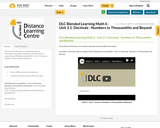Conditional Remix & Share Permitted
CC BY-NC-SA
Rating
0.0 stars

The purpose of this lesson is to explore decimals to thousandths and smaller.

Included is a YouTube video to support Grade 6 Blended Learning Math - Unit 3.1: Decimals - Numbers to Thousandths and Beyond.

Subject:
Math
Material Type:
Activity/Lab
Homework/Assignment
Lesson
Provider:
Sun West Distance Learning Centre (DLC)
05/16/2019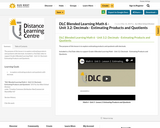Conditional Remix & Share Permitted
CC BY-NC-SA
Rating
0.0 stars

The purpose of this lesson is to explore estimating products and quotients with decimals.

Included is a YouTube video to support Grade 6 Blended Learning Math - Unit 3.2: Decimals - Estimating Products and Quotients.

Subject:
Math
Material Type:
Activity/Lab
Homework/Assignment
Lesson
Provider:
Sun West Distance Learning Centre (DLC)
05/16/2019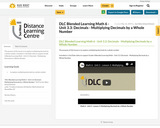Conditional Remix & Share Permitted
CC BY-NC-SA
Rating
0.0 stars

The purpose of this lesson is to explore multiplying decimals by a whole number.

Included is a YouTube video to support Grade 6 Blended Learning Math - Unit 3.3: Decimals - Multiplying Decimals by a Whole Number.

Subject:
Math
Material Type:
Activity/Lab
Homework/Assignment
Lesson
Provider:
Sun West Distance Learning Centre (DLC)
05/16/2019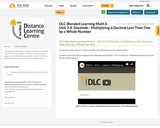Conditional Remix & Share Permitted
CC BY-NC-SA
Rating
0.0 stars

The purpose of this lesson is to explore multiply a decimal less than one by a whole number.

Included is a YouTube video to support Grade 6 Blended Learning Math - Unit 3.4: Decimals - Multiplying a Decimal Less Than One by a Whole Number.

Subject:
Math
Material Type:
Activity/Lab
Homework/Assignment
Lesson
Provider:
Sun West Distance Learning Centre (DLC)
05/16/2019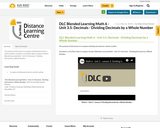Conditional Remix & Share Permitted
CC BY-NC-SA
Rating
0.0 stars

The purpose of this lesson is to explore dividing decimals by a whole number.

Included is a YouTube video to support Grade 6 Blended Learning Math - Unit 3.5: Decimals - Dividing Decimals by a Whole Number.

Subject:
Math
Material Type:
Activity/Lab
Homework/Assignment
Lesson
Provider:
Sun West Distance Learning Centre (DLC)
05/16/2019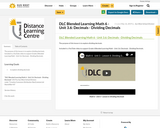Conditional Remix & Share Permitted
CC BY-NC-SA
Rating
0.0 stars

The purpose of this lesson is to explore dividing decimals.

Included is a YouTube video to support Grade 6 Blended Learning Math - Unit 3.6: Decimals - Dividing Decimals.

Subject:
Math
Material Type:
Activity/Lab
Homework/Assignment
Lesson
Provider:
Sun West Distance Learning Centre (DLC)
05/16/2019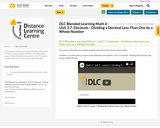Conditional Remix & Share Permitted
CC BY-NC-SA
Rating
0.0 stars

The purpose of this lesson is to explore dividing a decimal less than one by a whole number.

Included is a YouTube video to support Grade 6 Blended Learning Math - Unit 3.7: Decimals - Dividing a Decimal Less Than One by a Whole Number.

Subject:
Math
Material Type:
Activity/Lab
Homework/Assignment
Lesson
Provider:
Sun West Distance Learning Centre (DLC)
05/16/2019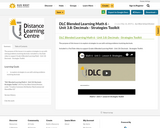Conditional Remix & Share Permitted
CC BY-NC-SA
Rating
0.0 stars

The purpose of this lesson is to explore strategies to use with solving problems involving decimals.

Included is a YouTube video to support Grade 6 Blended Learning Math - Unit 3.8: Decimals - Strategies Toolkit.

Subject:
Math
Material Type:
Activity/Lab
Homework/Assignment
Lesson
Provider:
Sun West Distance Learning Centre (DLC)
05/16/2019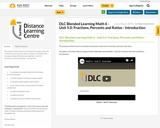Conditional Remix & Share Permitted
CC BY-NC-SA
Rating
0.0 stars

The purpose of this lesson is to introduce the grade 6 math unit on fractions, percents and ratios.

Included is a YouTube video to support Grade 6 Blended Learning Math - Unit 5.0: Fractions, Percents and Ratios - Introduction.

Subject:
Math
Material Type:
Activity/Lab
Homework/Assignment
Lesson
Provider:
Sun West Distance Learning Centre (DLC)